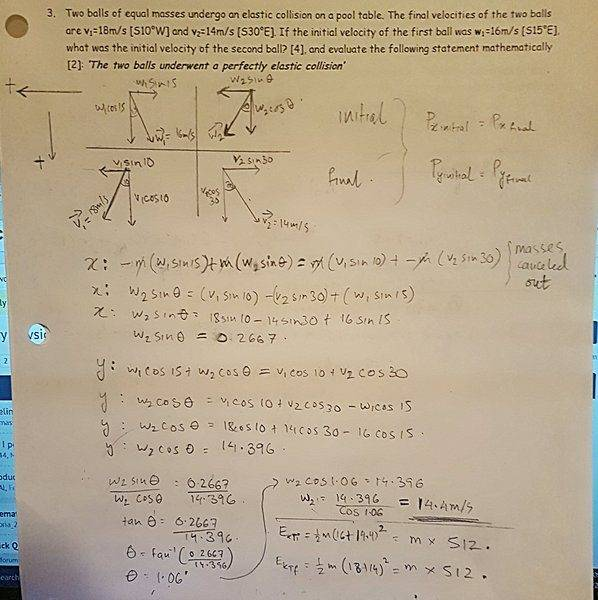# Question about Momentum and Collisions

## Homework Statement

a)Two balls of equal masses undergo and elastic collision on a pool table. The final velocities of the two balls are v1=18m/s [S10W] and v2= 14m/s [S30E]. If the initial velocity of the first ball was w1=16m/s [S15E], what was the initial velocity of the second ball?
b) Evaluate the following statement mathematically - "The two balls underwent a perfectly elastic collision."

p = mv

## The Attempt at a Solution

Firstly, I drew out all the given vectors in the questions. Initial velocities in the top half and final velocities in the bottom. Then I established reference directions. I realise that according to the conservation of momentum, the momentum will be equal initially and finally. I apply this to the x and y components of the vectors to form two seperate equations. By solving for the unknown velocity component in each case, I go onto equate them, allowing me to find the value of Theta.

After that, finally, I find the speed. I am not exacly sure how to deal with the last part of the question. Can anyone help to verify my work as well as provide any suggestions to the last part? Help would be very much appreciated!#### Attachments

Think about conservation rules do they apply to all situations? If you can, also think of collisions of equal masses and what is the special feature of that problem is it applicable here. your answer and your mistake is hidden in the observations I have suggested.

haruspex
Homework Helper
Gold Member
2020 Award
I get the feeling that the question setter is not using the correct definition of "elastic collision". In the standard definition there is no distinction between "elastic" and "perfectly elastic". Both mean the mechanical energy is conserved. But in that case the conditions are overspecified and inconsistent.

If any mechanical energy is lost it is inelastic; if the maximum possible is lost (subject to momentum conservation) it is perfectly inelastic.

Or maybe it is just a typo, the questioner meant to type "inelastic".

I get the feeling that the question setter is not using the correct definition of "elastic collision". In the standard definition there is no distinction between "elastic" and "perfectly elastic". Both mean the mechanical energy is conserved. But in that case the conditions are overspecified and inconsistent.

If any mechanical energy is lost it is inelastic; if the maximum possible is lost (subject to momentum conservation) it is perfectly inelastic.

Or maybe it is just a typo, the questioner meant to type "inelastic".

True. Questioner has not given you that. The questioner wants you to find out what is it.

I get the feeling that the question setter is not using the correct definition of "elastic collision". In the standard definition there is no distinction between "elastic" and "perfectly elastic". Both mean the mechanical energy is conserved. But in that case the conditions are overspecified and inconsistent.

If any mechanical energy is lost it is inelastic; if the maximum possible is lost (subject to momentum conservation) it is perfectly inelastic.

Or maybe it is just a typo, the questioner meant to type "inelastic".

You are partly right normally elastic means perfectly elastic. So there is some error in the statement of the problem. You can point it out by attacking the problem correctly. First apply that which is always true no matter what kind of collision it is. Then verify. It is simple. The adjective "elastic" does not need qualification in physics. The adjective "inelastic" needs further qualification of perfectly inelastic. It is obvious the collision is not perfectly inelastic.

Last edited: